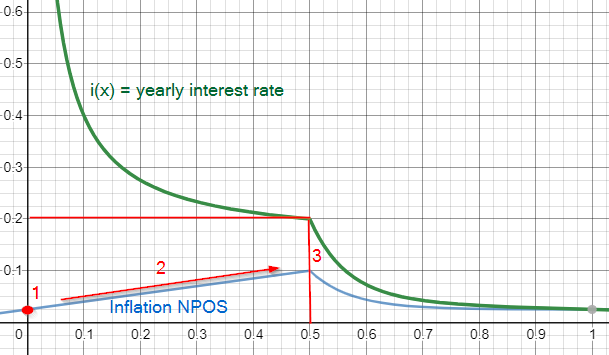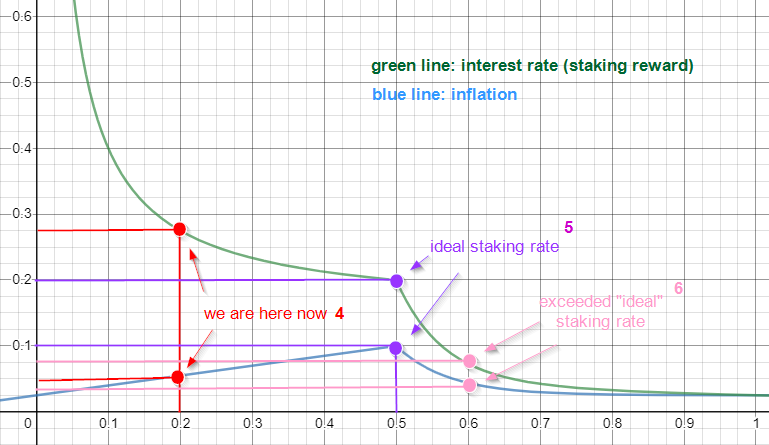# Intro

We are currently in the first phase of Polkadot’s launch. During this phase, called the “PoA-Phase”, ICO contributors can claim their DOTs and intend to nominate. In the next phase, after the switch from PoA-Phase to the NPOS-Phase, all validators and nominators will participate in the block production/validation and therefore be compensated with staking rewards. In this article we’ll look into how staking rewards are computed. Especially in the first few months the staking rewards are very high and it’s therefore very lucrative to put them at stake (through nomination). But before we go through some numbers out of nowhere lets take a look at the current economics.

## Important variables to know:

• Total supply: 10,000,000 DOTs
• Inflation: is designed to be 10% in the first year, and the ideal staking ratio is set at 50%, meaning half of all tokens should be locked in staking. In other words, if 50% of all tokens are staked, then 100% of the inflation goes to the validators as reward. If the staking rate is greater than or less than 50%, then the validators will receive less, with the remainder going to the Treasury.
• Inflation to validators: dynamic → depending on staking rate
• Claimed DOTs: ~2,500,000
• DOTs in staking: ~500,000
• Current staking rate: ~20% → (DOTs in staking / Claimed DOTs)*100

The third and fourth parameter changes daily, since more and more people claim and nominate their DOTs. Check the latest numbers here.

# Reward Table

This is the staking reward that you as a validator or nominator can expect. It is based on the token economics paper of Polkadot. Inflation is designed to be 10% (Inflation to Stakers + Inflation to Treasury) in the first year, with validator rewards being a function of amount staked and the remainder going to treasury.

If you want to understand how to compute the staking reward or how the formula is working continue reading. This is the inflation and interest rate formula that we’ll look into in the next two sections.

# Inflation & Staking Rate Formula

The value of the staking rate should lie between 30% and 60% - ideally at 50%. If it falls, the security is compromised, so we should give strong incentives to DOT holders to stake more of their assets. If it rises, we lose liquidity, so we should decrease the incentives sharply.

Now to the interesting part of this article: Below is a plot of the token economics formula that can be found here. But since both formulas are expressed in this plot, it is sufficient to understand the plot.

x = staking rate(x is always a value between 0 and 1)
green graph = i (x) yearly interest rate
blue graph =
I (x) inflation rate to stakersx-axis represents the total staking rate, y-axis interest rate (green) or inflation to validator rate (blue)

1 → Number 1 displayed in the graph is the minimum of the inflation to stakers (i.e. when neither validators nor nominators are staking DOTs). It’s the inflation “starting” point; 0.025 → 2.5% in this case goes away for validator rewards and the other 7.5% of the 10% inflation rate goes to the Polkadot treasury.

2 → The second number shows a linear increase of inflation if staking rate is between 0 and 50%. There is a linear correlation between staking rate and inflation till 0.5 is reached. Inflation rate is 5% if staking rate is at 25%. If staking rate doubles to 50%, so does the inflation rate to 10%. But remember: It is only linear till 50% staking rate is reached. In this ideal scenario the whole inflation moves to validators and nothing to the treasury.

3 → The red line at number 3 is the ideal staking rate of 50%. In this case, the annual staking reward is at 20%. But if the staking rates exceeds 50%, there is an exponential decay in inflation, which results in a strong decay of staking rewards. The reason for that is that the network needs liquidity and decreases the incentives sharply if the staking rate exceeds 50%. The difference of the 10% as always goes to the treasury.

If it’s not clear yet, don’t worry and look at the next graph. It might give you a better understanding.

# How High is the Staking Reward to this Date?

In this plot you see three main scenarios. The first one shows you where we are as of today (hypothetical — since NPOS is not active yet), what the ideal scenario is and the result of an exceeded “ideal” staking rate.x-axis represents the total staking rate, y-axis interest rate (green) or inflation validator rate (blue)

4 → Number 4 shows us where we are as of today. The current staking rate is at ~0.2 (20%) → (DOTs in staking / Claimed DOTs)*100). This means that we can, assuming NPoS (second phase of the launch process) is activated, expect a yearly interest rate of 27.5%. Yes, it’s an exponential decay so you won’t see such high rewards again. That’s why you should support the network too and gain some extra DOTs in doing so - especially in such a profitable period. You don’t know how to stake? Here’s a guide.

Validators share 5% of the 10% inflation, while the other half goes to the treasury.

5 → Take a look at the ideal scenario now. As already mentioned above, in this case the staking rate is at the ideal point. Validators get the whole 10% inflation, with yearly interest rate at 20%. Nothing goes to treasury.

6 → As soon as the staking rate exceeds 50%, inflation and therefore staking rewards decrease sharply. Why? To provide more liquidity through less incentives to stake. Let’s assume that the staking rate is at 60%, then the yearly interest rate will be at around 7.5% (exponential decay after 50% staking rate reached). Inflation for validators should be under 5% then and like always, the difference going to the treasury to fund further research and development.

You can find the plot here if you want to take a closer look.

# Conclusion

The token economics of Polkadot is very well thought out. Early stakers have a strong incentives to stake or even stake more, since the reward for it is exponential. You’ll never make higher staking reward on Polkadot than in the first six months after the activation of NPOS. It’s a unique chance to maximize your return by nominating validators. If you have any questions about the inflation or staking rewards join the official telegram channel: https://t.me/PolkadotOfficial or ping us directly https://t.me/swiss_staking.

## Nominate to Swiss Staking

If you haven’t nominated yet, please do. We would be more than happy if you choose us - Swiss Staking - as your validator. Swiss Staking is “Staking-as-a-Service” provider based in Switzerland. As one of the first validators on Tezos, one of 30 validators on the Alexander Polkadot Testnet and currently also in the validator set of Cosmos we have already gained over 700 days of staking experience.

• 13WAmUbyS4PMcPQe7bLXJdcuuF3A4cshY7QBGA94dzP2VxLk (0% fee), index of this address is: 1kLUd

--

--